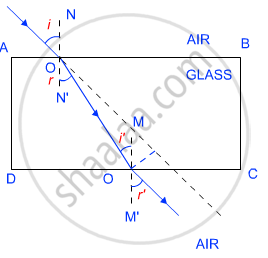# Refraction of Light

#### notes

REFRACTION:

The change of direction of light because of change of medium is known as Refraction or Refraction of Light. The ray of light changes its direction or phenomenon of refraction takes place because of difference in speed in different media.

The light travels at faster speed in rare medium and at slower speed in denser medium. The nature of media is taken as relative. For example air is a rarer medium than water or glass.

When ray of light enters from a rarer medium into a denser medium, it bends towards normal at the point of incidence. On the contrary, when ray of light enters into a rarer medium from a denser medium it bends away from the normal.

Ray emerging after the denser medium goes in the same direction and parallel to the incident ray.

The angle between incident ray and normal is called Angle of Incidence and it is denoted as ‘i’. The angle between refracted ray and normal is called the Angle of Refraction. Angle of refraction is denoted by ‘r’.Laws Of Refraction Of Light.

(i) The incident ray, the refracted ray and the normal to the interface of two transparent media at the point of incidence, all lie in the same plane.

(ii) The ratio of sine of angle of incidence to the sine of angle of refraction is a constant, for the light of a given colour and for the given pair of media. This law is also known as Snell’s law of refraction. (This is true for angle 0 < i < 90o) If i is the angle of incidence and r is the angle of refraction, then,

"sinI"/"sinr" = constant

This constant value is called the refractive index of the second medium with respect to the first.

If you would like to contribute notes or other learning material, please submit them using the button below.